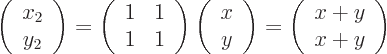#### 2.6.2 So­lu­tion herm-b

Ques­tion:

A ma­trixis de­fined to con­vert any vec­tor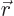into the vec­tor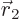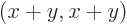. Ver­ify thatandare or­tho­nor­mal eigen­vec­tors of this ma­trix, with eigen­val­ues 2 re­spec­tively 0. Note: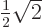.

An­swer:

For,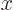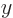so, and that is twice. For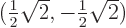,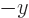so(0,0), and that is zero times.

The square length ofis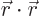, which is given by the sum of the square com­po­nents: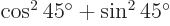. That is one, so the vec­tor is of length one. The same for. The dot prod­uct ofandis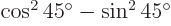. That is zero, be­cause, so the two eigen­vec­tors are or­thog­o­nal.

In lin­ear al­ge­bra, you would write the re­la­tion­ship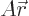out as: